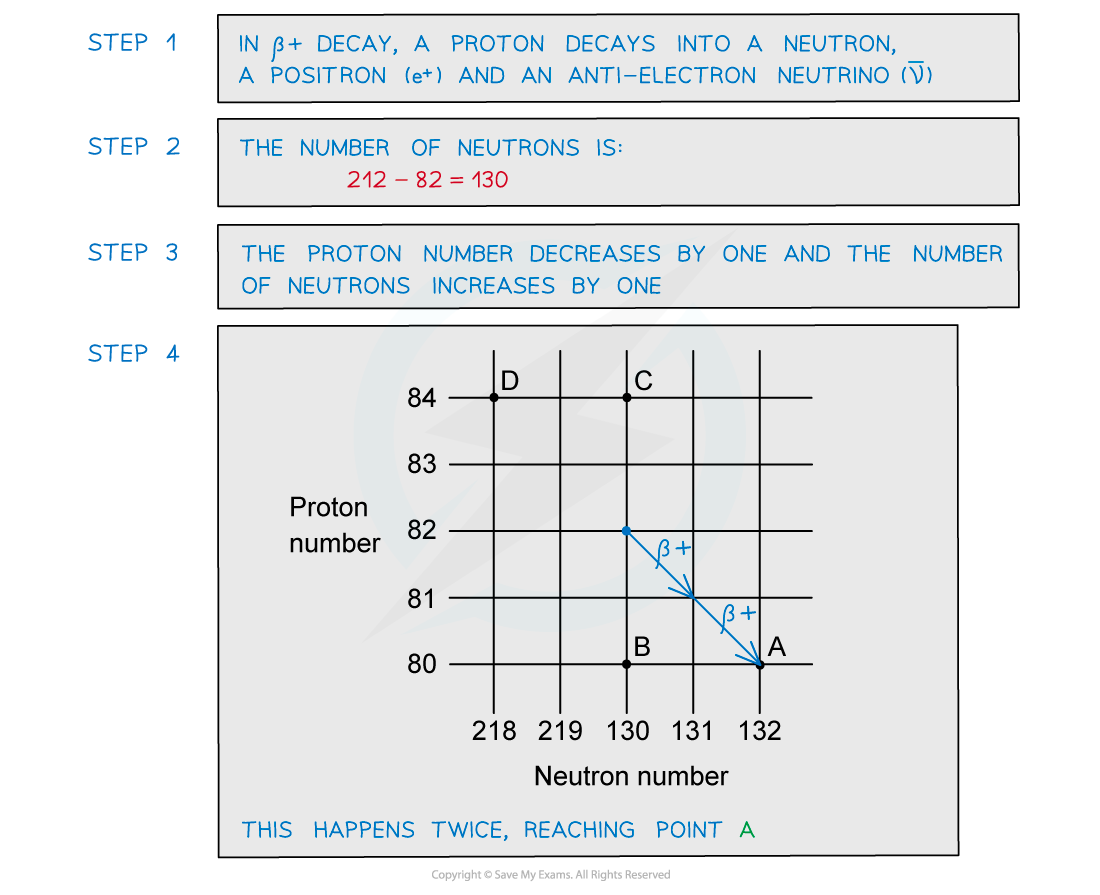# IB DP Physics: HL复习笔记7.1.7 Decay Equations

### Decay Equations

#### Changes in N and Z by Radioactive Decay

• There are four reasons why a nucleus might become unstable, and these determine which decay mode will occur
• Decays through beta-minus (β-) emission
• One of the neutrons in the nucleus changes into a proton and a β- particle (an electron) and antineutrino is released
• The nucleon number is constant
• The neutron number (N) decreases by 1
• The proton number (Z) increases by 1
• The general decay equation for β- emission is:Too many neutrons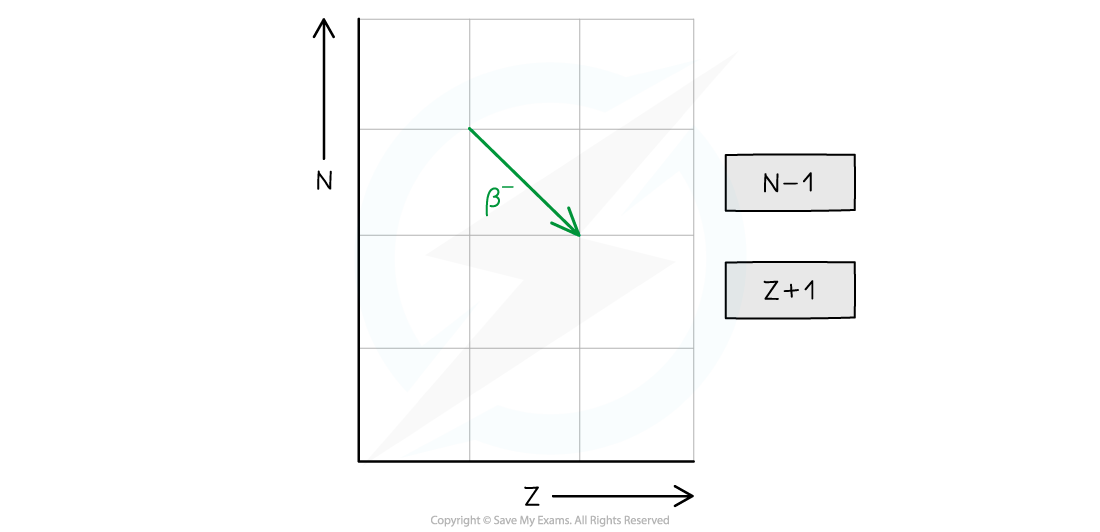• Too many protons
• Decays through beta-plus (β+) emission or electron capture
• In beta-plus decay, a proton changes into a neutron and a β+ particle (a positron) and neutrino are released
• In electron capture, an orbiting electron is taken in by the nucleus and combined with a proton causing the formation of a neutron and neutrino
• In both types of decay, the nucleon number stays constant
• The neutron number (N) increases by 1
• The proton number (Z) decreases by 1
• The general decay equation for β+ emission is:• The equation for electron capture is: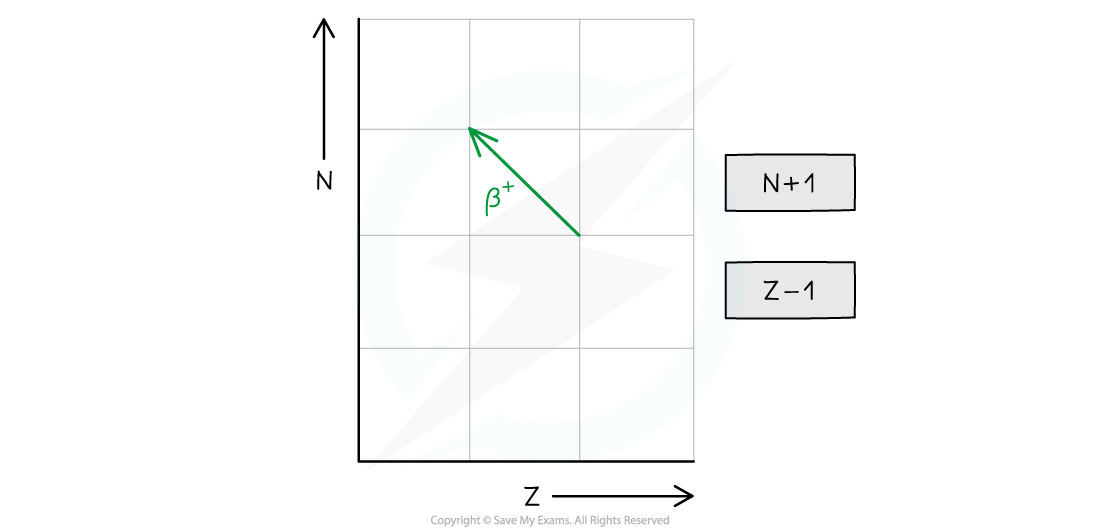• Too many nucleons
• Decays through alpha (α) emission
• An α particle is a helium nucleus
• The nucleon number decreases by 4 and the proton number decreases by 2
• The neutron number (N) decreases by 2
• The proton number (Z) decreases by 2
• The general decay equation for α emission is: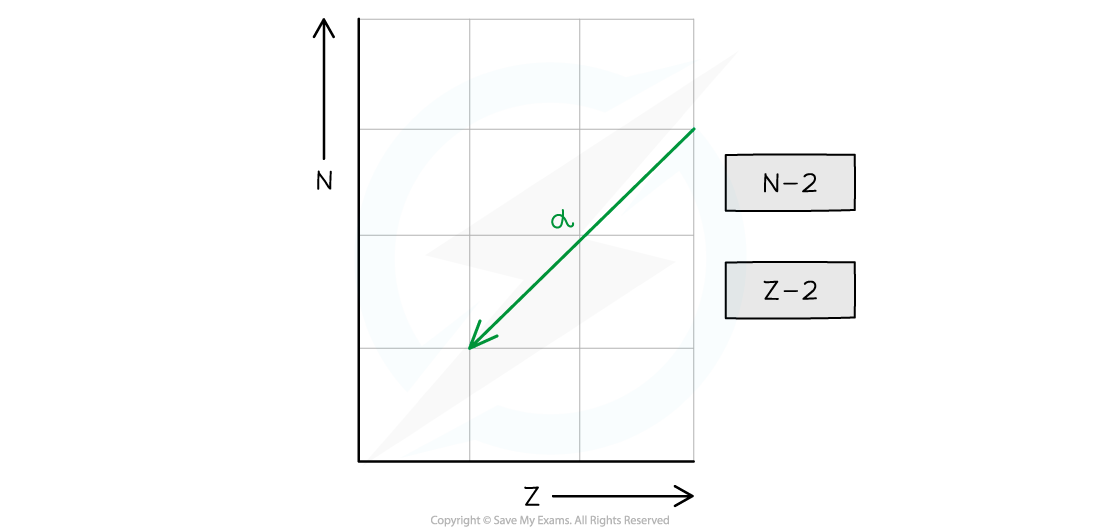• Too much energy
• Decays through gamma (γ) emission
• A gamma particle is a high-energy electromagnetic radiation
• This usually occurs after a different type of decay, such as alpha or beta decay
• This is because the nucleus becomes excited and has excess energy
• In summary, alpha decay, beta decay and electron capture can be represented on an N–Z graph as follows: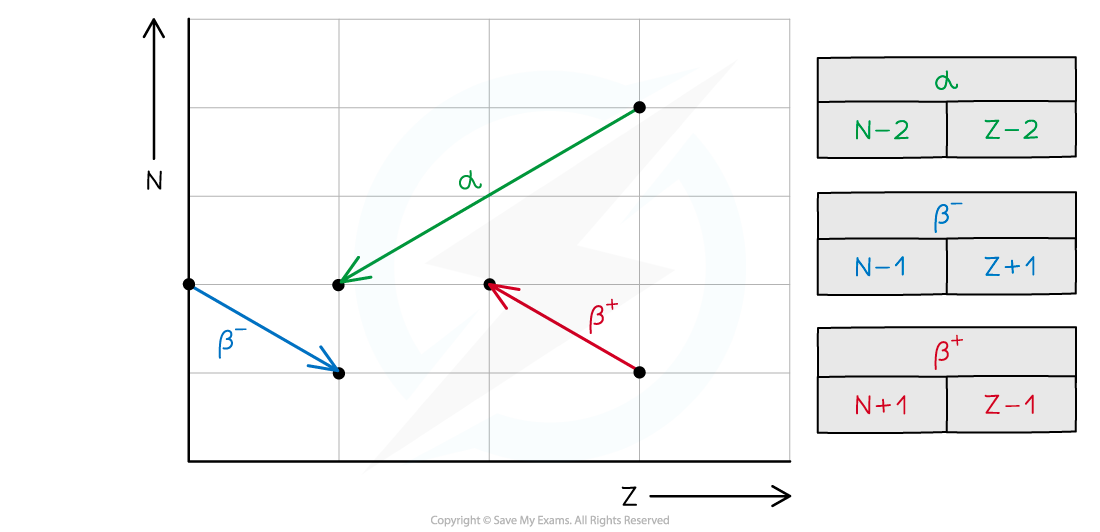#### Worked Example

A nucleus with 84 protons and 126 neutrons undergoes alpha decay. It forms lead, which has the element symbol Pb.Which of the isotopes of lead pictured is the correct one formed during the decay?

Step 1: Calculate the mass number of the original nucleus

• The mass number is equal to the number of protons plus the number of neutrons
• The original nucleus has 84 protons and 126 neutrons

84 + 126 = 210

• The mass number of the original nucleus is 210

Step 2: Calculate the new atomic number

• The alpha particle emitted is made of two protons and two neutrons
• Protons have an atomic number of 1, and neutrons have an atomic number of 0
• Removing two protons and two neutrons will reduce the atomic number by 2

84 – 2 = 82

• The new nucleus has an atomic number of 82

Step 3: Calculate the new mass number

• Protons and neutrons both have a mass number of 1
• Removing two protons and two neutrons will reduce the mass number by 4

210 – 4 = 206

• The new nucleus has a mass number of 206

#### Worked Example

Plutonium-239 is a radioactive isotope that contains 94 protons and emits α particles to form a radioactive isotope of uranium. This isotope of uranium emits α particles to form an isotope of thorium which is also radioactive.

Write two equations to represent the decay of plutonium-239 and the subsequent decay of uranium.

Step 1: Write down the general equation of alpha decayStep 2: Write down the decay equation of plutonium into uraniumStep 3: Write down the decay equation of uranium into thorium#### Worked Example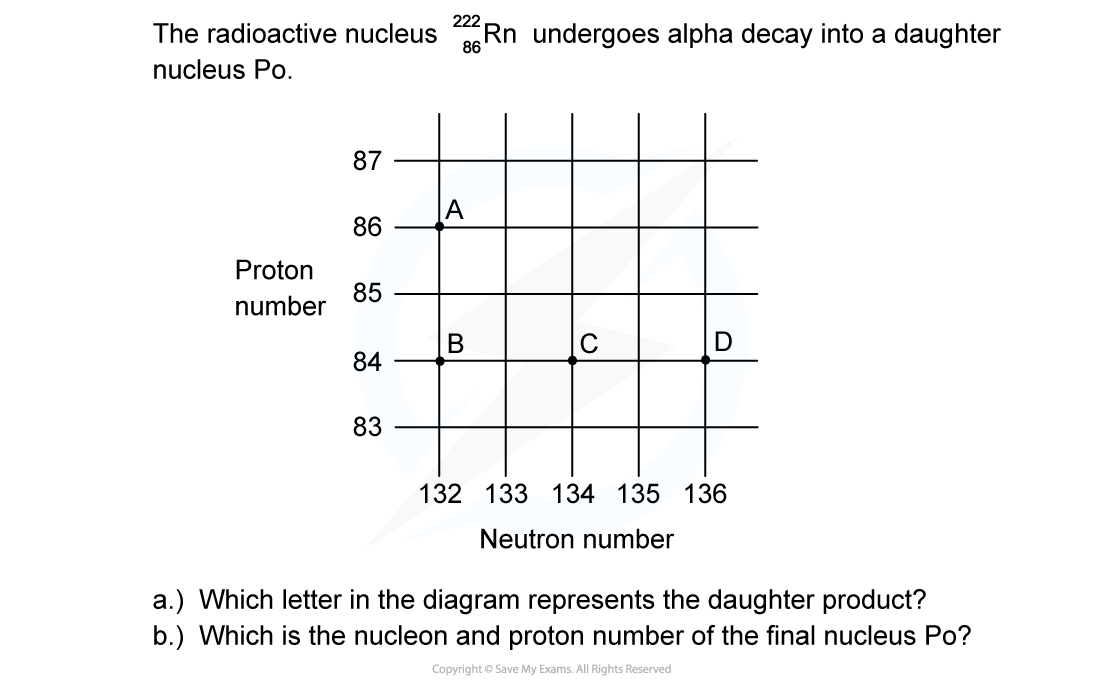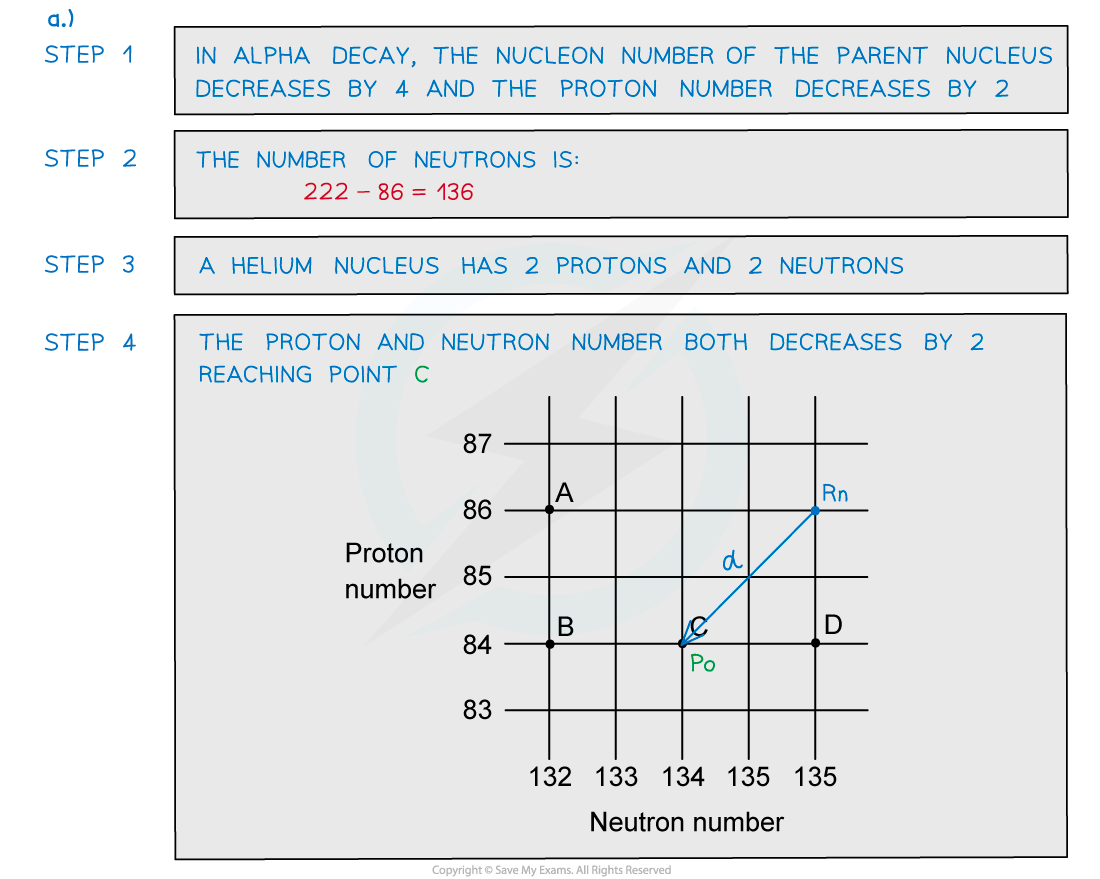#### Worked Example

A radioactive substance with a nucleon number of 212 and a proton number of 82 decays by β-plus emission into a daughter product which in turn decays by further β-plus emission into a granddaughter product.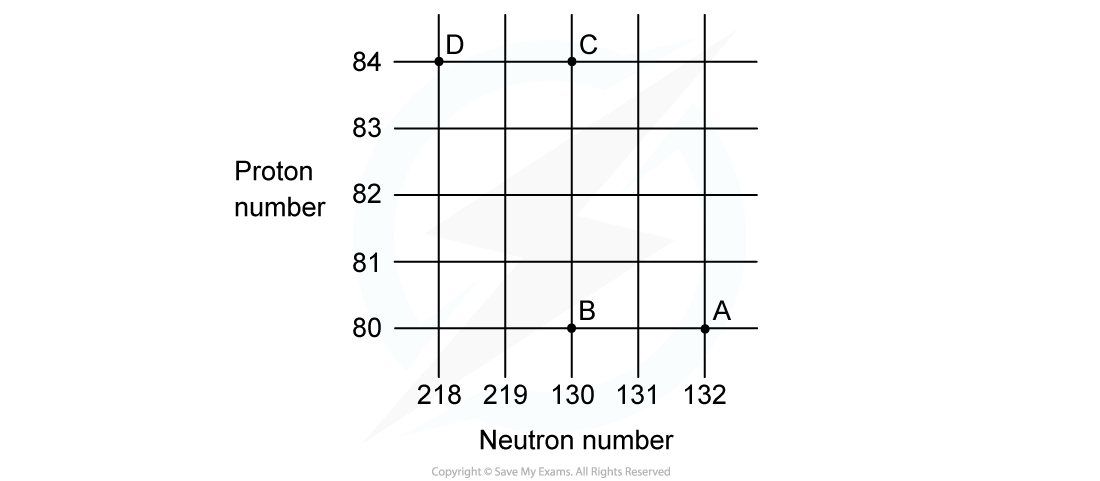Which letter in the diagram represents the granddaughter product?

ANSWER: A